Latest Banking jobs   »   Quantitative Aptitude Quiz For SBI Clerk...

# Quantitative Aptitude Quiz For SBI Clerk Prelims 2022- 4th May

Q1. By selling two articles a man earns 15% profit on first article and 10% loss on second article. Find his overall gain or loss percent if C.P. of both articles were same?
(a) 2%
(b) 5%
(c) 2.5%
(d) 3%
(e) 3.5%

Q2. A shopkeeper in place of selling a article at 10% profit, mark up the article 30% above cost price and gives a discount of 10%. In this process he gets Rs. 56 more profit. Find the cost price of article.
(a) Rs. 400
(b) Rs. 1000
(c) Rs. 800
(d) Rs. 600
(e) Rs. 500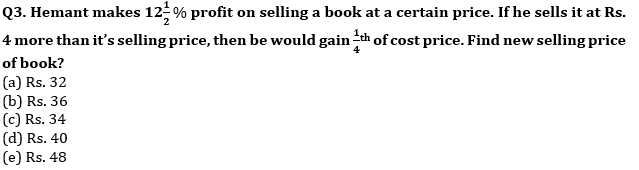Q4. Manoj invests Rs. 1800 in two parts at SI at 4% and x% for two years. When he invests larger part at x% and smaller part at 4% then he gets total of Rs. 164 as interest and when he invests larger part at 4% and smaller part at x% then he gets total of Rs. 160 as interest. Find value of x%?
(a) 5%
(b) 6%
(c) 3%
(d) 7%
(e) None of these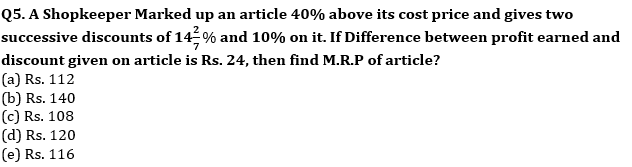Q6. Manoj invested a sum at x% per annum at C.I. If first year and second year C.I on that sum is Rs. 845 and Rs. 910 Find Amount invested?
(a) Rs. 10985
(b) Rs. 10000
(c) Rs. 13000
(d) Rs. 10900
(e) Rs. 13985

Q7. Dinesh started a business with investing Rs 12000 and after some months, Sunny joined with investing Rs 9000. At the end of the year, total profit was Rs 8000 and share of Sunny is Rs 1600. Find after how many months did sunny joined in the business?
(a) 6 months
(b) 4 months
(c) 9 months
(d) 3 months
(e) 8 months

Q8. Two horses were sold at Rs 12600. First horse was sold at 20% loss and second at 25% profit. If the cost price of first horse is equal to selling price of second horse. Find the overall profit/loss.
(a) Rs 360
(b) Rs 440
(c) No profit or no loss
(d) Rs 542
(e) Can’t be determined

Q9. If the compound interest on a certain sum for 2 years is Rs 308 and simple interest at same rate of interest and for same time is 280. Find the sum?
(a) Rs 700
(b) Rs 1200
(c) Rs 1100
(d) Rs 500
(e) Rs 900

Q10. Lokesh marks up his goods 20% above cost price and allows 25% discount on them while selling. if he sells the article for Rs 1080, then what was the cost price?
(a) Rs 1200
(b) Rs 1080
(c) Rs 1134
(d) Rs 1120
(e) Rs 1300

Q11. A invested Rs.2000 and B invested Rs.500 more than A. After 8 months C invested Rs.1500. At the end of the year C got profit of Rs.350. Find total profit.
(a) Rs.3500
(b) Rs.4200
(c) Rs.2800
(d) Rs.4900
(e) Rs.2100

Q12. Selling price of an article becomes Rs.1080 after giving two successive discounts of x% and 25% and marked price of article is Rs.1800. Find the cost price of article if there is a profit of x% on selling the article after giving two successive discounts.
(a) Rs.860
(b) Rs.750
(c) Rs.800
(d) Rs.900
(e) Rs.850

Q13. Akash invested Rs 48000 at the rate of 15% per annum for one year. If the interest is compounded half-yearly, then find the amount received by Akash after one year.
(a) Rs 58470
(b) Rs 47470
(c) Rs 55470
(d) Rs 45470
(e) Rs 44570

Q14. A started a business with an investment of Rs 54000 and after some months, B joined him with investing Rs 45000. At the end of the year, total profit was Rs 35700 and share of A in the total profit is Rs 22950. Find after how many months B has joined the business.
(a) 5 months
(b) 6 months
(c) 4 months
(d) 2 months
(e) 3 months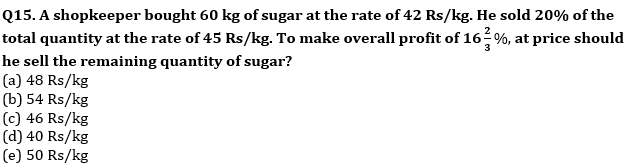Solutions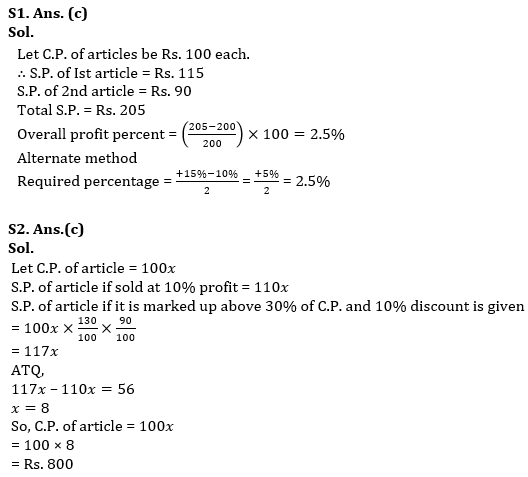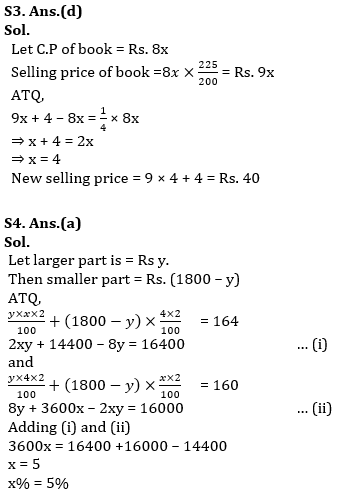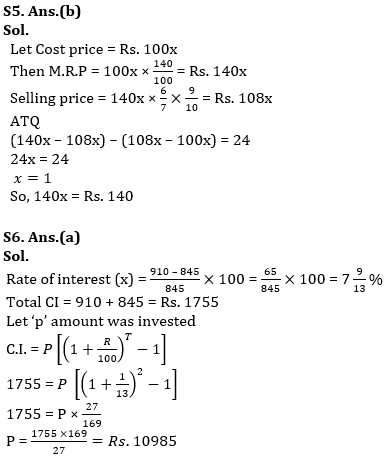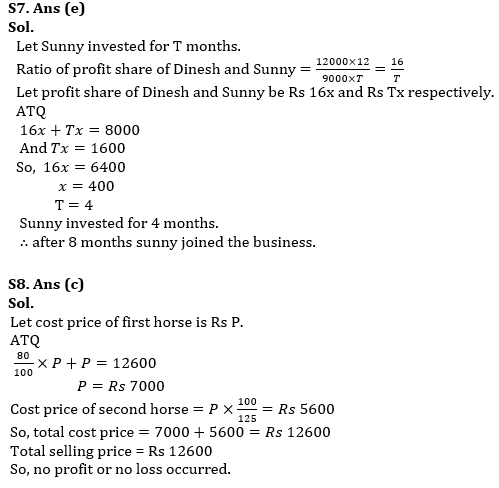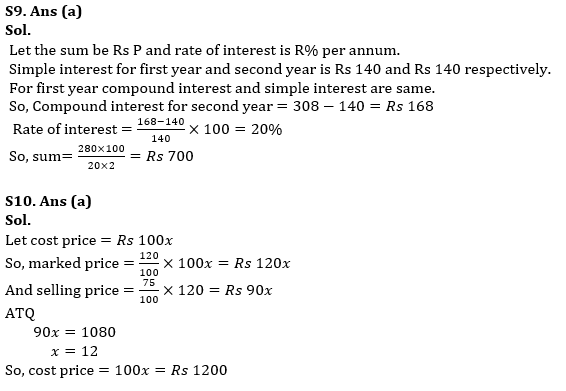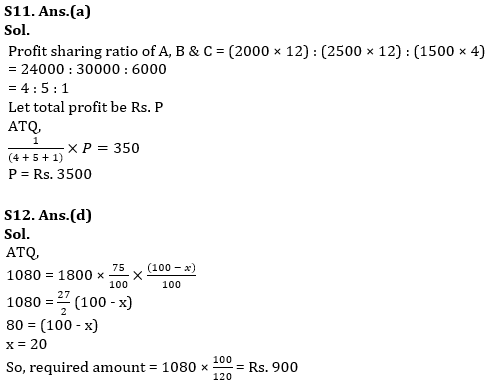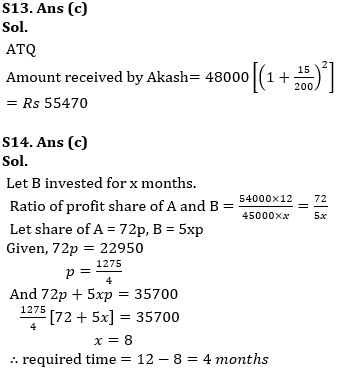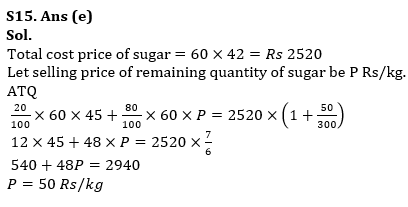#### Congratulations!

Incorrect details? Fill the form again here

•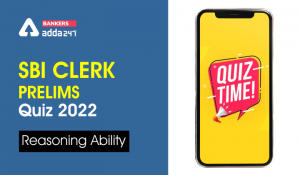Reasoning Ability Quiz For SBI Clerk Pre...
•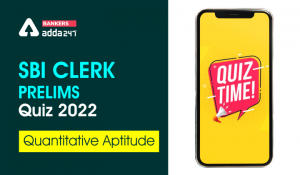Quantitative Aptitude Quiz For SBI Clerk...
•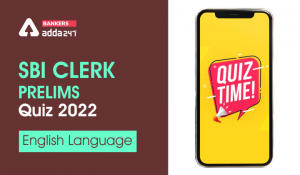English Quizzes For SBI Clerk Prelims 20...
•Quantitative Aptitude Quiz For SBI Clerk...
•English Quizzes For SBI Clerk Prelims 20...
•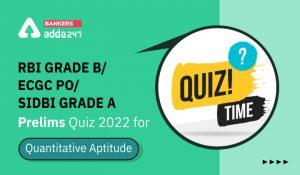Quantitative Aptitude Quiz For RBI Grade...